Balbharti 12th Maharashtra State Board Maths Solutions Book Pdf Chapter 5 Vectors Ex 5.4 Questions and Answers.

## Maharashtra State Board 12th Maths Solutions Chapter 5 Vectors Ex 5.4

Question 1.
If $$\bar{a}$$ = $$2 \hat{i}+3 \hat{j}-\hat{k}$$, $$\bar{b}$$ = $$\hat{i}-4 \hat{j}+2 \hat{k}$$ find ($$\bar{a}$$ + $$\bar{b}$$) × ($$\bar{a}$$ – $$\bar{b}$$)
Solution: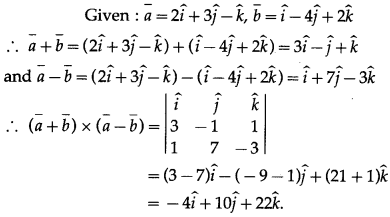Question 2.
Find a unit vector perpendicular to the vectors $$\hat{j}+2 \hat{k}$$ and $$\hat{i}+\hat{j}$$.
Solution:
Let $$\bar{a}$$ = $$\hat{j}+2 \hat{k}$$, $$\bar{b}$$ = $$\hat{i}+\hat{j}$$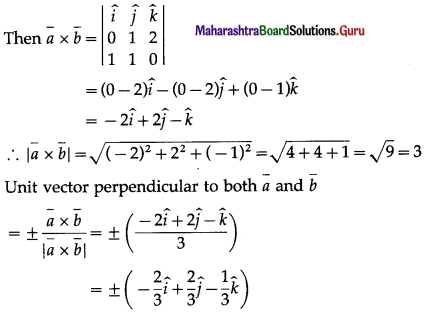Question 3.
If $$\bar{a} \cdot \bar{b}$$ = $$\sqrt {3}$$ and $$\bar{a} \times \bar{b}$$ = $$2 \hat{i}+\hat{j}+2 \hat{k}$$, find the angle between $$\bar{a}$$ and $$\bar{b}$$.
Solution:
Let θ be the angle between $$\bar{a}$$ and $$\bar{b}$$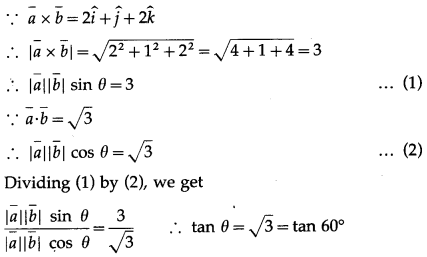∴ θ = 60°.Question 4.
If $$\bar{a}$$ = $$2 \hat{i}+\hat{j}-3 \hat{k}$$ and $$\bar{b}$$ = $$\hat{i}-2 \hat{j}+\hat{k}$$, find a vector of magnitude 5 perpendicular to both $$\bar{a}$$ and $$\bar{b}$$.
Solution:
Given : $$\bar{a}$$ = $$2 \hat{i}+\hat{j}-3 \hat{k}$$ and $$\bar{b}$$ = $$\hat{i}-2 \hat{j}+\hat{k}$$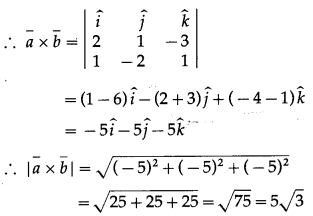∴ unit vectors perpendicular to both the vectors $$\bar{a}$$ and $$\bar{b}$$.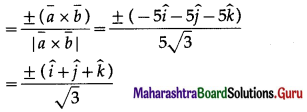∴ required vectors of magnitude 5 units
= ±$$\frac{5}{\sqrt{3}}(\hat{i}+\hat{j}+\hat{k})$$

Question 5.
Find
(i) $$\bar{u}$$∙$$\bar{v}$$ if $$|\bar{u}|$$ = 2, $$|\vec{v}|$$ = 5, $$|\bar{u} \times \bar{v}|$$ = 8
Solution:
Let θ be the angle between $$\bar{u}$$ and $$\bar{v}$$.
Then $$|\bar{u} \times \bar{v}|$$ = 8 gives
$$|\bar{u}||\bar{v}|$$ sin θ = 8
∴ 2 × 5 × sin θ = 8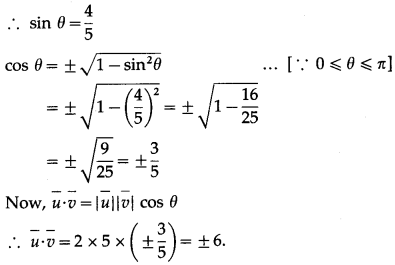(ii) $$|\bar{u} \times \bar{v}|$$ if $$|\bar{u}|$$ = 10, $$|\vec{v}|$$ = 2, $$\bar{u} \cdot \bar{v}$$ = 12
Solution:
Let θ be the angle between $$\bar{u}$$ and $$\bar{v}$$.
Then $$\bar{u} \cdot \bar{v}$$ = 12 gives
$$|\bar{u} \| \bar{v}|$$cos θ = 12
∴ 10 × 2 × cos θ = 12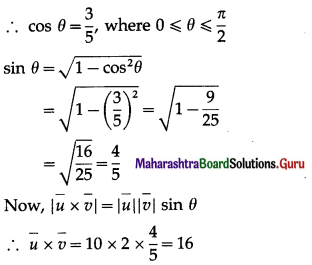Question 6.
Prove that 2($$\bar{a}$$ – $$\bar{b}$$) × 2($$\bar{a}$$ + $$\bar{b}$$) = 8($$\bar{a}$$ × $$\bar{b}$$)
Solution: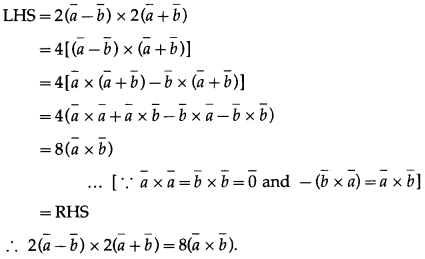Question 7.
If $$\bar{a}$$ = $$\hat{i}-2 \hat{j}+3 \hat{k}$$, $$\bar{b}$$ = $$4 \hat{i}-3 \hat{j}+\hat{k}$$, and $$\bar{c}$$ = $$\hat{i}-\hat{j}+2 \hat{k}$$, verify that $$\bar{a}$$ × ($$\bar{b}$$ + $$\bar{c}$$) = $$\bar{a}$$ × $$\bar{b}$$ + $$\bar{a}$$ × $$\bar{c}$$
Solution:
Given : $$\bar{a}$$ = $$\hat{i}-2 \hat{j}+3 \hat{k}$$, $$\bar{b}$$ = $$4 \hat{i}-3 \hat{j}+\hat{k}$$, $$\bar{c}$$ = $$\hat{i}-\hat{j}+2 \hat{k}$$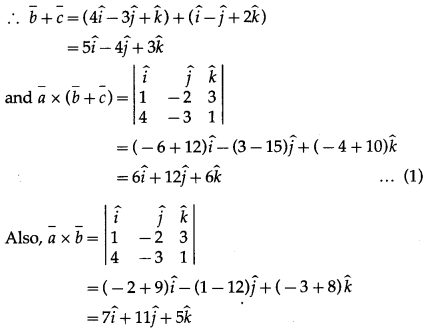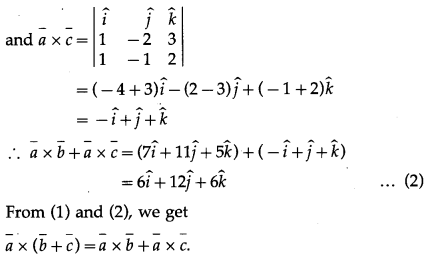Question 8.
Find the area of the parallelogram whose adjacent sides are the vectors $$\bar{a}$$ = $$2 \hat{i}-2 \hat{j}+\hat{k}$$ and $$\bar{b}$$ = $$\hat{i}-3 \hat{j}-3 \hat{k}$$.
Solution:
Given : $$\bar{a}$$ = $$2 \hat{i}-2 \hat{j}+\hat{k}$$, $$\bar{b}$$ = $$\hat{i}-3 \hat{j}-3 \hat{k}$$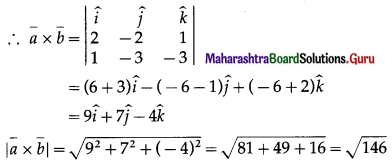Area of the parallelogram whose adjacent sides are $$\bar{a}$$ and $$\bar{b}$$ is $$|\bar{a} \times \bar{b}|$$ =$$\sqrt {146}$$ sq units.Question 9.
Show that vector area of a quadrilateral ABCD is $$\frac{1}{2}$$ ($$\overline{A C}$$ × $$\overline{B D}$$), where AC and BD are its diagonals.
Solution:
Let ABCD be a parallelogram.
Then $$\overline{\mathrm{AC}}$$ = $$\overline{\mathrm{AB}}$$ + $$\overline{\mathrm{BC}}$$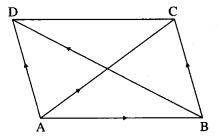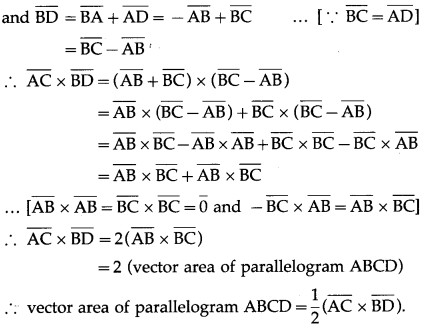Question 10.
Find the area of parallelogram whose diagonals are determined by the vectors $$\bar{a}$$ = $$3 i-\hat{j}-2 \hat{k}$$, and $$\bar{b}$$ = $$-\hat{i}+3 \hat{j}-3 \hat{k}$$
Solution:
Given: $$\bar{a}$$ = $$3 i-\hat{j}-2 \hat{k}$$, $$\bar{b}$$ = $$-\hat{i}+3 \hat{j}-3 \hat{k}$$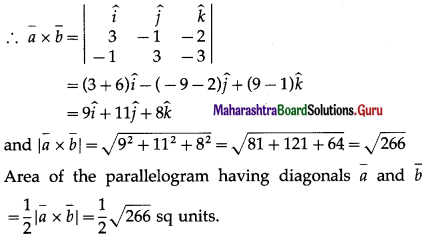Question 11.
If $$\bar{a}$$, $$\bar{b}$$, $$\bar{c}$$ and $$\bar{d}$$ are four distinct vectors such that $$\bar{a} \times \bar{b}=\bar{c} \times \bar{d}$$ and $$\bar{a} \times \bar{c}=\bar{b} \times \bar{d}$$, prove that $$\bar{a}$$ – $$\bar{d}$$ is parallel to $$\bar{b}$$ – $$\bar{c}$$.
Solution:
$$\bar{a}$$, $$\bar{b}$$, $$\bar{c}$$ and $$\bar{d}$$ are four distinct vectors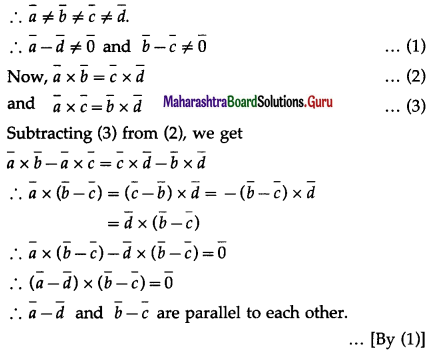Question 12.
If $$\bar{a}$$ = $$\hat{i}+\hat{j}+\hat{k}$$ and, $$\bar{c}$$ = $$\hat{j}-\hat{k}$$, find a vector $$\bar{b}$$ satisfying $$\bar{a}$$ × $$\bar{b}$$ = $$\bar{c}$$ and $$\bar{a} \cdot \bar{b}$$ = 3
Solution:
Given $$\bar{a}$$ = $$\hat{i}+\hat{j}+\hat{k}$$, $$\bar{c}$$ = $$\hat{j}-\hat{k}$$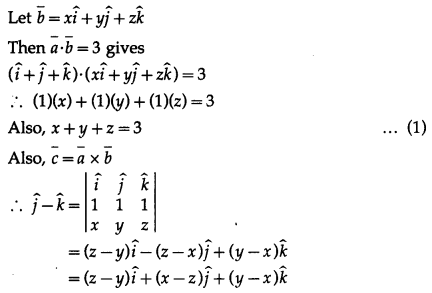By equality of vectors,
z – y = 0 ….(2)
x – z = 1 ……(3)
y – x = -1 ……(4)
From (2), y = z.
From (3), x = 1 + z
Substituting these values of x and y in (1), we get
1 + z + z + z = 3 ∴ z = $$\frac{2}{3}$$
∴ y = z = $$\frac{2}{3}$$
∴ x = 1 + z =1 + $$\frac{2}{3}=\frac{5}{3}$$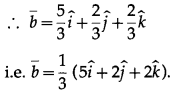Question 13.
Find $$\bar{a}$$, if $$\bar{a} \times \hat{i}+2 \bar{a}-5 \hat{j}=\overline{0}$$.
Solution: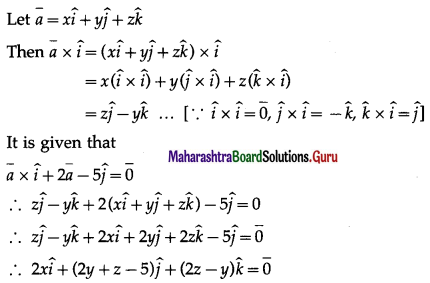By equality of vectors
2x = 0 i.e. x = 0
2y + z – 5 = 0 … (1)
2z – y = 0 … (2)
From (2), y = 2z
Substituting y = 2z in (1), we get
4z + z = 5 ∴ z = 1
∴ y = 2z = 2(1) = 2
∴ x = 0, y = 2, z = 1
∴ $$\bar{a}=2 \hat{j}+\hat{k}$$

Question 14.
If $$|\bar{a} \cdot \bar{b}|$$ = $$|\bar{a} \times \bar{b}|$$ and $$\bar{a} \cdot \bar{b}$$ < 0, then find the angle between $$\bar{a}$$ and $$\bar{b}$$
Solution:
Let θ be the angle between $$\bar{a}$$ and $$\bar{b}$$.
Then $$|\bar{a} \cdot \bar{b}|$$ = $$|\bar{a} \times \bar{b}|$$ gives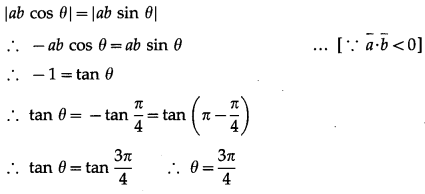Hence, the angle between $$\bar{a}$$ and $$\bar{b}$$ is $$\frac{3 \pi}{4}$$.Question 15.
Prove by vector method that sin (α + β) = sinα∙cosβ+cosα∙sinβ.
Solution: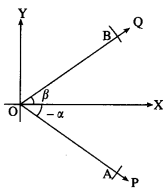Let ∠XOP and ∠XOQ be in standard position and m∠XOP = -α, m∠XOQ = β.
Take a point A on ray OP and a point B on ray OQ such that
OA = OB = 1.
Since cos (-α) = cos α
and sin (-α) = -sin α,
A is (cos (-α), sin (-α)),
i.e. (cos α, – sin α)
B is (cos β, sin β)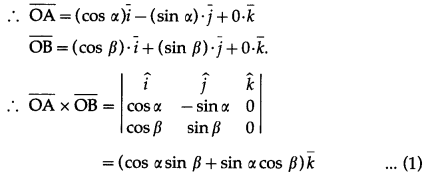The angle between $$\overline{\mathrm{OA}}$$ and $$\overline{\mathrm{OB}}$$ is α + β.
Also $$\overline{\mathrm{OA}}$$, $$\overline{\mathrm{OB}}$$ lie in the XY-plane.
∴ the unit vector perpendicular to $$\overline{\mathrm{OA}}$$ and $$\overline{\mathrm{OB}}$$ is $$\bar{k}$$.
∴ $$\overline{\mathrm{OA}}$$ × $$\overline{\mathrm{OB}}$$ = [OA∙OB sin (α + β)]$$\bar{k}$$
= sin(α + β)∙$$\bar{k}$$ …(2)
∴ from (1) and (2),
sin (α + β) = sin α cos β + cos α sin β.

Question 16.
Find the direction ratios of a vector perpendicular to the two lines whose direction ratios are
(i) -2, 1, -1 and -3, -4, 1
Solution:
Let a, b, c be the direction ratios of the vector which is perpendicular to the two lines whose direction ratios are -2, 1, -1 and -3, -4, 1
∴ -2a + b – c = 0 and -3a – 4b + c = 0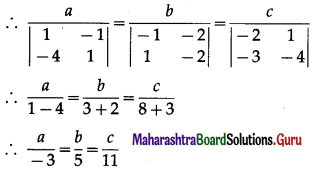∴ the required direction ratios are -3, 5, 11
Alternative Method:
Let $$\bar{a}$$ and $$\bar{b}$$ be the vectors along the lines whose direction ratios are -2, 1, -1 and -3, -4, 1 respectively.
Then $$\bar{a}$$ = $$-2 \hat{i}+\hat{j}-\hat{k}$$ and $$\bar{b}$$ = $$-3 \hat{i}-4 \hat{j}+\hat{k}$$
The vector perpendicular to both $$\bar{a}$$ and $$\bar{b}$$ is given by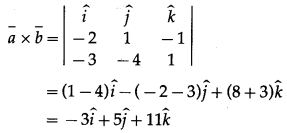Hence, the required direction ratios are -3, 5, 11.

(ii) 1, 3, 2 and -1, 1, 2
Solution:Question 17.
Prove that two vectors whose direction cosines are given by relations al + bm + cn = 0 and fmn + gnl + hlm = 0 are perpendicular if $$\frac{f}{a}+\frac{g}{b}+\frac{h}{c}$$ = 0
Solution:
Given, al + bm + cn = 0 …(1)
and fmn + gnl + hlm = 0 …..(2)
From (1), n = $$-\left(\frac{a l+b m}{c}\right)$$ …..(3)
Substituting this value of n in equation (2), we get
(fm + gl)∙$-\left(\frac{a l+b m}{c}\right)$ + hlm = 0
∴ -(aflm + bfm2 + agl2 + bglm) + chlm = 0
∴ agl2 + (af + bg – ch)lm + bfm2 = 0 … (4)
Note that both l and m cannot be zero, because if l = m = 0, then from (3), we get
n = 0, which is not possible as l2 + m2 + n2 = 1.
Let us take m # 0.
Dividing equation (4) by m2, we get
ag$$\left(\frac{l}{m}\right)^{2}$$ + (af + bg – ch)$$\left(\frac{l}{m}\right)$$ + bf = 0 … (5)
This is quadratic equation in $$\left(\frac{l}{m}\right)$$.
If l1, m1, n1 and l2, m2, n2 are the direction cosines of the two lines given by the equation (1) and (2), then $$\frac{l_{1}}{m_{1}}$$ and $$\frac{l_{2}}{m_{2}}$$ are the roots of the equation (5).
From the quadratic equation (5), we get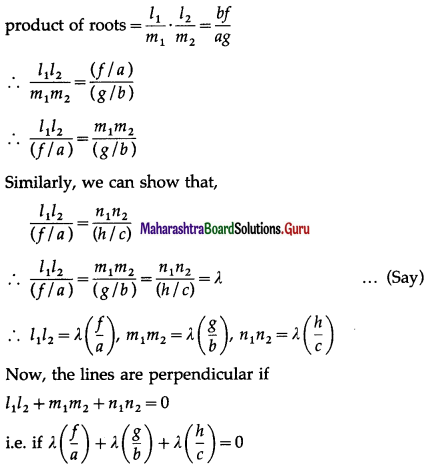i.e. if $$\frac{f}{a}+\frac{g}{b}+\frac{h}{c}$$ = 0.Question 18.
If A(1, 2, 3) and B(4, 5, 6) are two points, then find the foot of the perpendicular from the point B to the line joining the origin and point A.
Solution: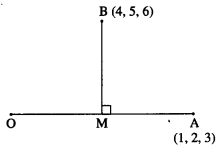Let M be the foot of the perpendicular drawn from B to the line joining O and A.
Let M = (x, y, z)
OM has direction ratios x – 0, y – 0, z – 0 = x, y, z
OA has direction ratios 1 – 0, 2 – 0, 3 – 0 = 1, 2, 3
But O, M, A are collinear.
∴ $$\frac{x}{1}=\frac{y}{2}=\frac{z}{3}$$ = k …(Let)
∴ x = k, y = 2k, z = 3k
∴ M = (k, 2k, 3k)
∵ BM has direction ratios
k – 4, 2k – 5, 3k – 6
BM is perpendicular to OA
∴ (l)(k – 4) + 2(2k – 5) + 3(3k – 6)
∴ = k – 4 + 4k – 10 + 9k – 18 = 0
∴ 14k = 32
∴ k = $$\frac{16}{7}$$
∴ M = (k, 2k, 3k) = ($$\frac{16}{7}, \frac{32}{7}, \frac{48}{7}$$)